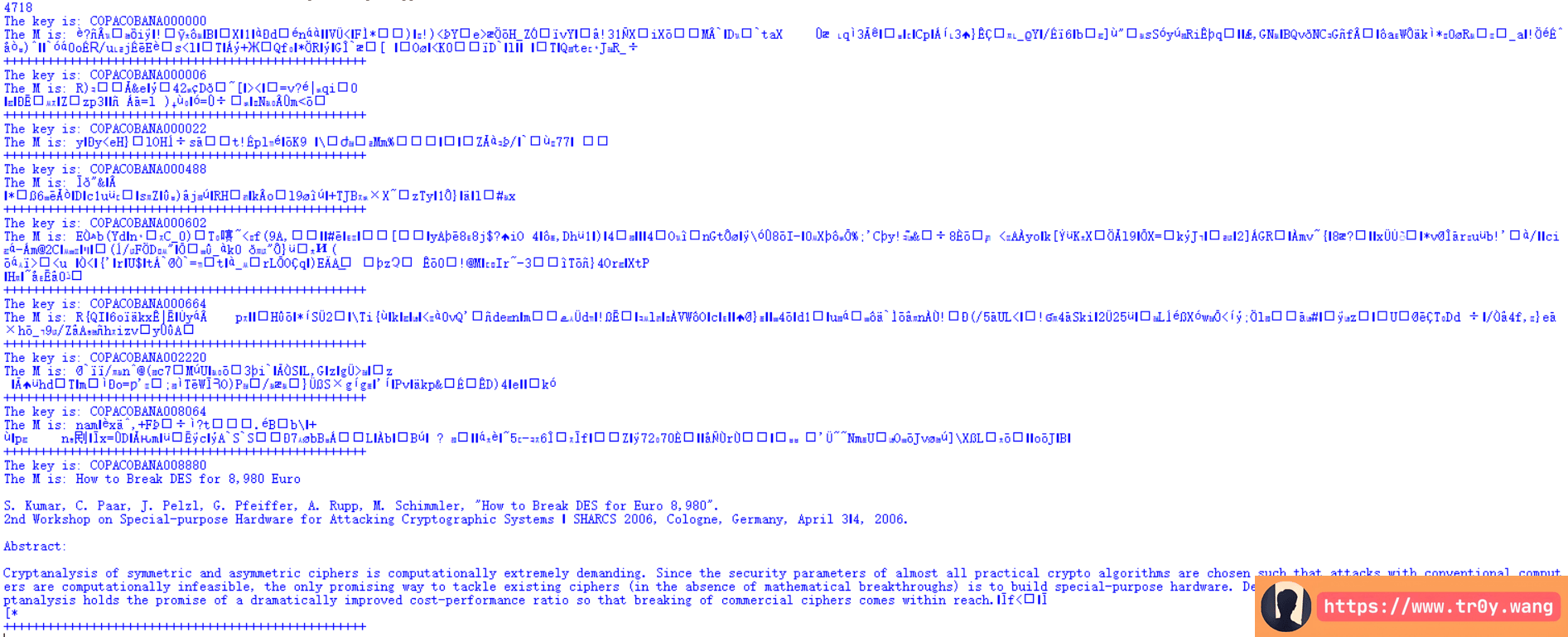Tr0y 10月 06, 2017 20:58:31 本文共 268 字
• 文为知己者书
•• 在其它设备中阅读本文章
•mysterytwisterc3 的一道 LVII 的题, 很简单
brute_force_attack_on_triple_des_with_reduced_key_space

key 的前几个字符给出了提示, 是在 2006 年发明用于攻击 DES 密文的暴力破解机器，这个机器攻击 DES key (56 Bit) 仅仅花费不到一周。

COPACOBANA

from Crypto.Cipher import DES3
import re

with open('1.txt','rb') as fp:
data = ''.join(fp.read().split())

print len(data)
N = 0
for k in range(999999):
iv = str(k).zfill(6)
key = 'COPACOBANA'+iv
cipher = DES3.new(key, DES3.MODE_CBC, IV=('0'*16).decode('hex'))
m = cipher.decrypt(data.decode('hex')[:2352])
n = len(re.findall('[a-zA-Z]',m))
if N < n:
N = n
M = m
K = key

print 'The key is:',K
print 'The M is:',M
print '+'*50End

What do you think?